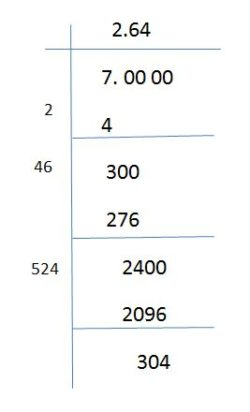# Square Root of 7

In Mathematics, the square root of 7 is a number, which is multiplied by itself for two times results in the original number 7. To find the square root of the given number, we need to know if it is a perfect square or not. Number 7 is not a perfect square. Therefore, we have to find the square root of 7 using the long division method.  In this article, we are going to learn how to find the value of square root 7 using the long division method with complete explanation.

## What is the Value of Square Root of 7?

The value of square root of 7 is a number which when multiplied by itself two times results in the original number. Symbolically, the square root of 7 is expressed as √7. In other words, the radical form of square root of 7 is √7.

√7 = √(Number × Number)

Thus, if we multiply 2.645 two times, we obtain the number 7.

(i.e) √7 = √(2.645×2.645)

√7 = √(2.645)2

Now, cancel out square and square root, we get

√7 = 2.645.

Thus, the square root of 7, √7 is approximately equal to 2.645.

 Square Root of 7 in Decimal Form: 2. 645

## Square Root of 7 by Long Division Method

Since the number 7 is not a perfect square number, the square root of 7 is found using the long division method. Now, follow the below steps to find the value of √7.

1. First, write the number 7 in decimal form. (i.e) 7. 0000
2. Now, divide the number 7 by a number such the product of same number should be smaller than or equal to 7. Thus, 2×2 = 4, which is smaller than 7.
3. Now, subtract 4 from 7, we will get the remainder 3 and quotient 2.
4. Now, double the quotient and we get 4 and assume that 40 is the new divisor.
5. Bring down two zeros and we get 300 is our new dividend.
6. Now, find the number, such that (40+ new number)×new number should be less than or equal to 300. Hence, (40+6)×6 = 276. Hence, 276 < 300.
7. Again, subtract 276 from 300. Hence, we get a remainder of 24 and the quotient is 26.
8. Repeat this same process until we get the value of square root of 7 up to 3 decimal places. (Put the decimal point in the quotient once the division process is completed).
9. Thus, the value of square root of 7 is approximately equal to 2.645.## Video Lessons on Square Roots

### Visualising square roots### Finding Square roots### Examples

Example 1:

Simplify the expression: 2√7 + 5√7.

Solution:

Given: 2√7 + 5√7.

2√7+5√7 = 7√7.

We know that √7 is approximately equal to 2.645.

Now, substitute the value, we get

2√7+5√7 = 7(2.645) = 18.515.

Example 2:

Find the value of a is a√7= 7.935.

Solution:

Given: a√7= 7.935

Thus, a = 7.935/√7

a = 7.935/2.645 [Since, √7 = 2.645]

a = 3

Hence, the value of a is 3.

Stay tuned with BYJU’S – The Learning App to learn more Maths-related articles and also watch engaging videos.

## Frequently Asked Questions on Square Root of 7

Q1

### What is the approximate value of square root of 7?

The value of square root of 7 is approximately equal to 2.645 (Rounded to 3 decimal places).

Q2

### Is √7 a rational or irrational number?

√7 is an irrational number, as the value of √7 cannot be expressed in the form of p/q.

Q3

### What is the value of square of square root of 7?

The square of square root of 7 is 7.
(i.e) (√7)2 = 7.

Q4

### Is 7 a perfect square number?

No, 7 is not a perfect square number.

Q5

### What is the value of 7 plus square root of 7?

We know that √7 = 2.645
Thus, 7+√7 = 7+2.645 = 9.645.

Test your Knowledge on Square Root of 7